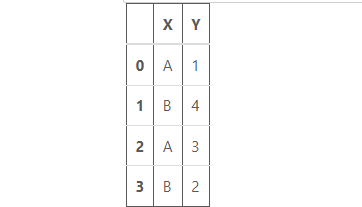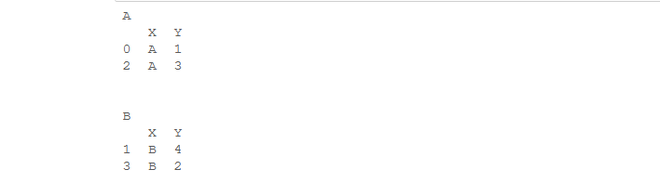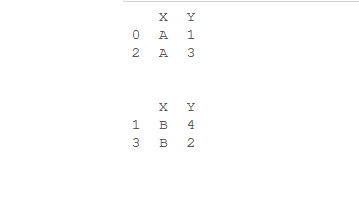Related Articles
How to Iterate over Dataframe Groups in Python-Pandas?
• Last Updated : 18 Aug, 2020

In this article, we’ll see how we can iterate over the groups in which a dataframe is divided. So, let’s see different ways to do this task.

First, Let’s create a dataframe:

Code:

## Python3

 `# import pandas library``import` `pandas as pd`` ` `# dictionary``dict` `=` `{``'X'``: [``'A'``, ``'B'``, ``'A'``, ``'B'``],``        ``'Y'``: [``1``, ``4``, ``3``, ``2``]}`` ` `# create a dataframe``df ``=` `pd.DataFrame(``dict``)`` ` `# show the dataframe``df`

Output:Method 1: Using Dataframe.groupby().

This function is used to split the data into groups based on some criteria.

Example: we’ll simply iterate over all the groups created.

## Python3

 `# import pandas library``import` `pandas as pd`` ` `# dictionary``dict` `=` `{``'X'``: [``'A'``, ``'B'``, ``'A'``, ``'B'``],``        ``'Y'``: [``1``, ``4``, ``3``, ``2``]}`` ` `# create a dataframe``df ``=` `pd.DataFrame(``dict``)`` ` `# group by 'X' column``groups ``=` `df.groupby(``"X"``)`` ` `for` `name, group ``in` `groups:``    ``print``(name)``    ``print``(group)``    ``print``(``"\n"``)`

Output:In above example, we have grouped on the basis of column “X”. As there are two different values under column “X”, so our dataframe will be divided into 2 groups. Then our for loop will run 2 times as the number groups are 2. “name” represents the group name and “group” represents the actual grouped dataframe.

Method 2: Using Dataframe.groupby() and Groupby_object.groups.keys() together.

Groupby_object.groups.keys() method will return the keys of the groups.

Example: we’ll iterate over the keys.

## Python3

 `# import pandas library``import` `pandas as pd`` ` `# dictionary``dict` `=` `{``'X'``: [``'A'``, ``'B'``, ``'A'``, ``'B'``],``        ``'Y'``: [``1``, ``4``, ``3``, ``2``]}`` ` `# create a dataframe``df ``=` `pd.DataFrame(``dict``)`` ` `# group by "X" column``groups ``=` `df.groupby(``'X'``)`` ` `# extract keys from groups``keys ``=` `groups.groups.keys()`` ` `for` `i ``in` `keys:``    ``print``(groups.get_group(i))``    ``print``(``'\n'``)`

Output:In above example, we’ll use the function groups.get_group() to get all the groups. First we’ll get all the keys of the group and then iterate through that and then calling get_group() method for each key. get_group()  method will return group corresponding to the key.

Attention geek! Strengthen your foundations with the Python Programming Foundation Course and learn the basics.

To begin with, your interview preparations Enhance your Data Structures concepts with the Python DS Course. And to begin with your Machine Learning Journey, join the Machine Learning – Basic Level Course

My Personal Notes arrow_drop_up